Naam Ben Stocker Functie DJ, producer Geslacht man Alias Ron Bacardi Lid van groepen Assailants & Frequency 7 Herkomst Verenigd KoninkrijkGenres techno Aanwezigheid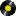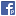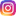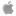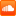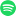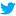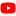Affiliaties 3

### Biografie

From early formative days earning his stripes spinning hip hop, house and rave on pirate radio, and at parties around Essex and East London, through the 90's as an ever more in demand DJ, and on into the new millennium as a label boss, producer and internationally recognised techno legend in his own right, Ben Sims has spent all his conscious life exploring all facets of underground dance music

-
Statistieken
-

## Video's

-

### 19 opmerkingen

ik heb een set van sims met hawtin Live @­ Electro tramp.....­tis house?! hele vette house! maar house?! iemand deze set ook geluisterd/ en heeft een mening?!
Ik kan het me haast niet voorstellen maar er zitten wel dingen in die me aan hawtin doen denken, en het is super strak gemixed...­
Martyro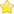Artiest Martyro
Uitspraak van verwijderd op donderdag 13 november 2008 om 08:18:
ik heb een set van sims met hawtin Live @­ Electro tramp.....­tis house?! hele vette house! maar house?! iemand deze set ook geluisterd/ en heeft een mening?!
Ik kan het me haast niet voorstellen maar er zitten wel dingen in die me aan hawtin doen denken, en het is super strak gemixed...­

die heb ik ook, heerlijk zomers set!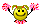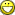het schijnt dat die set fake is, maar volgens mij qua datum. de set schijnt uit 2001 te komen
sims draait ook heel graag disco, funk en classics. en daar lijkt deze house met disco invloeden wel op. en ook hawtin zie ik hier wel voor aan

maar luister ook maar es een set van Essex Rascals (Ben Sims, Mark Broom, Paul Mac & Tony Anderson)
www.essexrascals.com
www.essexrascals.com/site/podcast/podcast.html
www.myspace.com/theessexrascals
laatste aanpassing
<3<3<3<3<3<3­<3<3<3<3<3<3­<3<3<3<3<3<3­<3<3<3<3<3<3­<3<3<3<3<3<3­<3<3<3<3<3<3­<3<3<3<3<3<3­<3<3<3<3<3<3­<3<3<3<3<3<3­<3<3<3<3<3<3­<3<3<3<3<3<3­<3<3<3<3<3<3­<3<3<3<3<3<3­<3<3<3<3<3<3­<3<3<3<3<3<3­<3<3<3<3<3<3­<3<3<3<3<3<3­<3<3<3<3<3<3­<3<3<3<3<3<3­<3<3<3<3<3<3­<3<3<3<3<3<3­<3<3<3<3<3<3­<3<3<3<3<3<3­<3<3<3<3<3<3­<3<3<3<3<3<3­<3<3<3<3<3<3­<3<3<3<3<3<3­<3<3<3<3<3<3­<3<3<3<3<3<3­<3<3<3<3<3<3­<3<3<3<3<3<3­<3<3<3<3<3<3­<3<3<3<3<3<3­<3<3<3<3<3<3­<3<3<3<3<3<3­<3<3<3<3<3<3­<3<3<3<3<3<3­<3<3<3<3<3<3­<3<3<3<3<3<3­<3<3<3<3<3<3­<3<3<3<3<3<3­<3<3<3<3<3<3­<3<3<3<3<3<3­<3<3<3<3<3<3­<3<3<3<3<3<3­<3<3<3<3<3<3­<3<3<3<3<3<3­<3<3<3<3<3<3­<3<3<3<3<3<3­<3<3<3<3<3<3­<3<3<3<3<3<3­<3<3<3<3<3<3­<3<3<3<3<3<3­<3<3<3<3<3<3­<3<3<3<3<3<3­<3<3<3<3<3<3­<3<3<3<3<3<3­<3<3<3<3<3<3­<3<3<3<3<3<3­<3<3<3<3<3<3­<3<3<3<3<3<3­<3<3<3